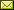## Propositional Logics Complexity and the Sub-Formula Property

 Edward Hermann Haeusler (PUC-Rio)

 In 1979 Richard Statman proved, using proof-theory, that the purely implicational fragment of Intuitionistic Logic (M-imply) is PSPACE-complete. He showed a polynomially bounded translation from full Intuitionistic Propositional Logic into its implicational fragment. By the PSPACE-completeness of S4, proved by Ladner, and the Goedel translation from S4 into Intuitionistic Logic, the PSPACE- completeness of M-imply is drawn. The sub-formula principle for a deductive system for a logic L states that whenever F1,...,Fk proves A, there is a proof in which each formula occurrence is either a sub-formula of A or of some of Fi. In this work we extend Statman result and show that any propositional (possibly modal) structural logic satisfying a particular formulation of the sub-formula principle is in PSPACE. If the logic includes the minimal purely implicational logic then it is PSPACE-complete. As a consequence, EXPTIME-complete propositional logics, such as PDL and the common-knowledge epistemic logic with at least 2 agents satisfy this particular sub-formula principle, if and only if, PSPACE=EXPTIME. We also show how our technique can be used to prove that any finitely many-valued logic has the set of its tautologies in PSPACE.

In Ugo Dal Lago and Russ Harmer: Proceedings Tenth International Workshop on Developments in Computational Models (DCM 2014), Vienna, Austria, 13th July 2014, Electronic Proceedings in Theoretical Computer Science 179, pp. 1–16.
Published: 10th April 2015.

 ArXived at: https://dx.doi.org/10.4204/EPTCS.179.1 bibtex PDF
References in reconstructed bibtex, XML and HTML format (approximated).
 Comments and questions to:eptcs@eptcs.org For website issues:webmaster@eptcs.org